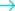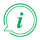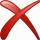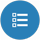# Convert power from Joule per second to Watt## Convert from Joule per second

### About Joule per second to Watt converter

##### Info

To convert from Joule per second to Watt fill in the conversion tool field with the amount you want to convert. The result of Joule per second to Watt conversion will be appeared in the "Result" field in red characters, without need of pressing any button. Below the conversion tool, a list will appear with all the available conversions from Joule per second.

#### Examples of Common Queries about converting Joule per second to Watt

##### Joule per second to Watt converter helps you to find a solution about:
• How do I turn Joule per second into Watt?
• How to convert Joule per second to Watt.
• How to make Joule per second Watt.
• How do I convert Joule per second power to Watt power ?
• Is Joule per second to Watt converter free?
• Where can i find Joule per second to Watt converter online.
• Is there a way to convert Joule per second to Watt?